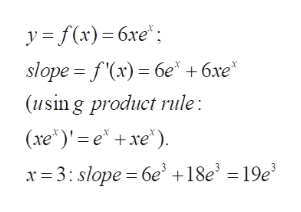# Find an equation for the line tangent to the graph of f(x) = 6xex at the point (a,f(a)) for a =3

Question
15 views

Find an equation for the line tangent to the graph of

f(x) = 6xex

at the point (a,f(a)) for a =3

check_circle

Step 1

To write down the equation of the tangent to the curve at the given point

Step 2

Recall: the tangent to the curve y=f(x) at (a,f(a) ) has slope f'(a) (the derivative evaluated at a) and of course passes through (a,f(a).

Step 3

calculation of the slope of...help_outlineImage Transcriptionclosey f(x) 6xe slope = f'(x) 6e" +6xe* (using product rule: (xeexe x= 3: slope = 6e +18e? = 19e fullscreen

### Want to see the full answer?

See Solution

#### Want to see this answer and more?

Solutions are written by subject experts who are available 24/7. Questions are typically answered within 1 hour.*

See Solution
*Response times may vary by subject and question.
Tagged in

### Derivative